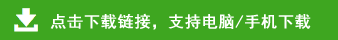# 小学四年级上册数学寒假作业

小学四年级上册数学寒假作业部分内容预览

一、计 算
一、直接写出得数
90÷15=       50×14=     120÷24=
20÷8=         105-70=    12×50=
180÷15=     360÷24=    160×5=
9×10+20=   25×8=      （24-18）÷6=
840÷60=      320÷64=    100-18+19=
320÷16-11=    70÷14=     15×7=
200÷25=         80-12×4=
二、口算下面各题的商是几
三、先试商，再算一算
四、在○里填运算符号，在□里填数，使等号两边式子的值相等。
五、根据商不变的性质，在□里填数
750÷150=75÷□     4600÷230=□÷23
96÷32=□÷1          8700÷300=□÷3
六、列竖式计算，打★要验算。
★408×76        340×56        168×85
★232÷29        551÷42       ★240÷16
七、递等式计算：
14×18-988÷13           414+486÷18-146
800-(325+720÷24)     (335-20) ÷(117÷13)
35×［（47+118）÷55］    ［750-（98+85）］÷3